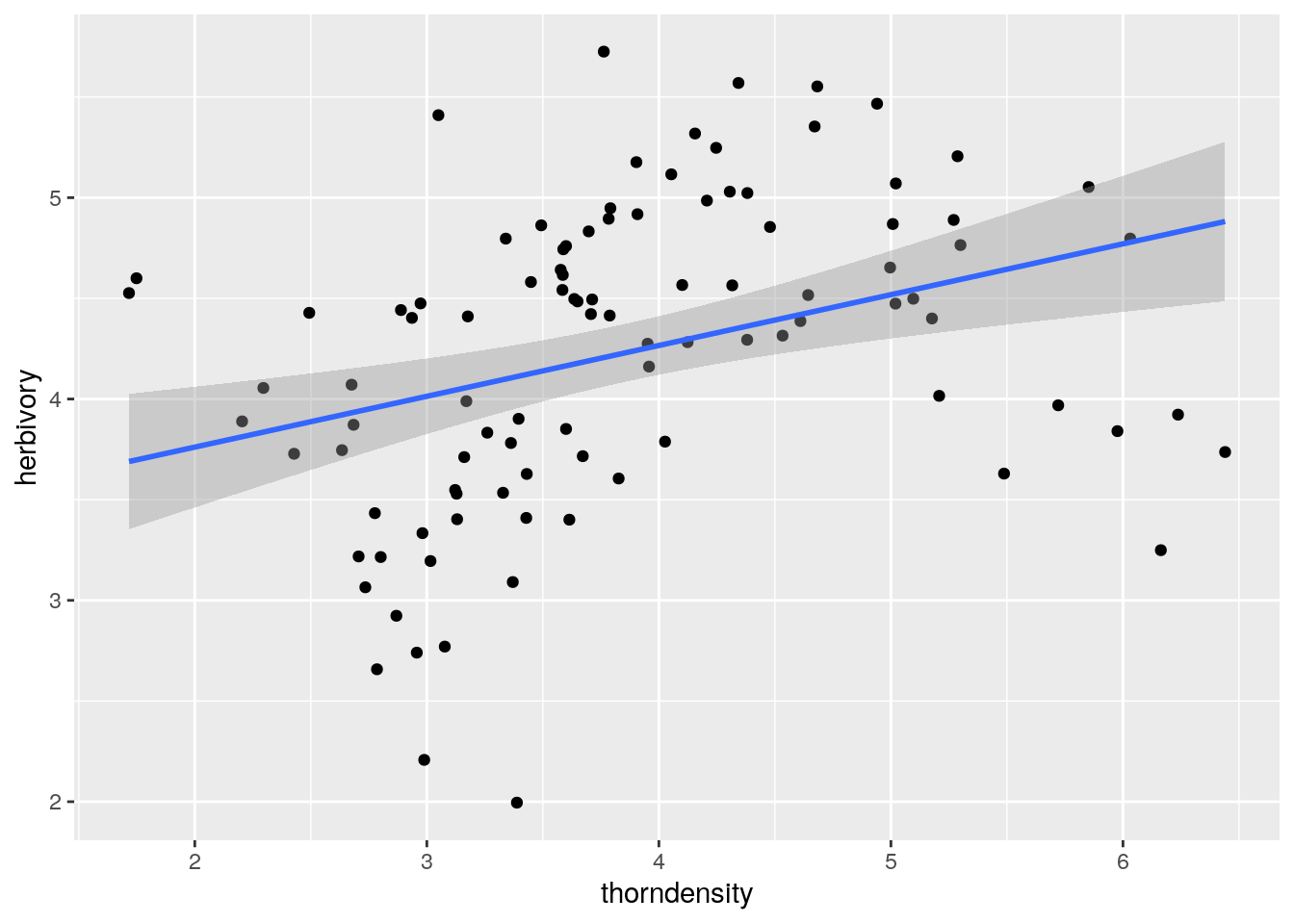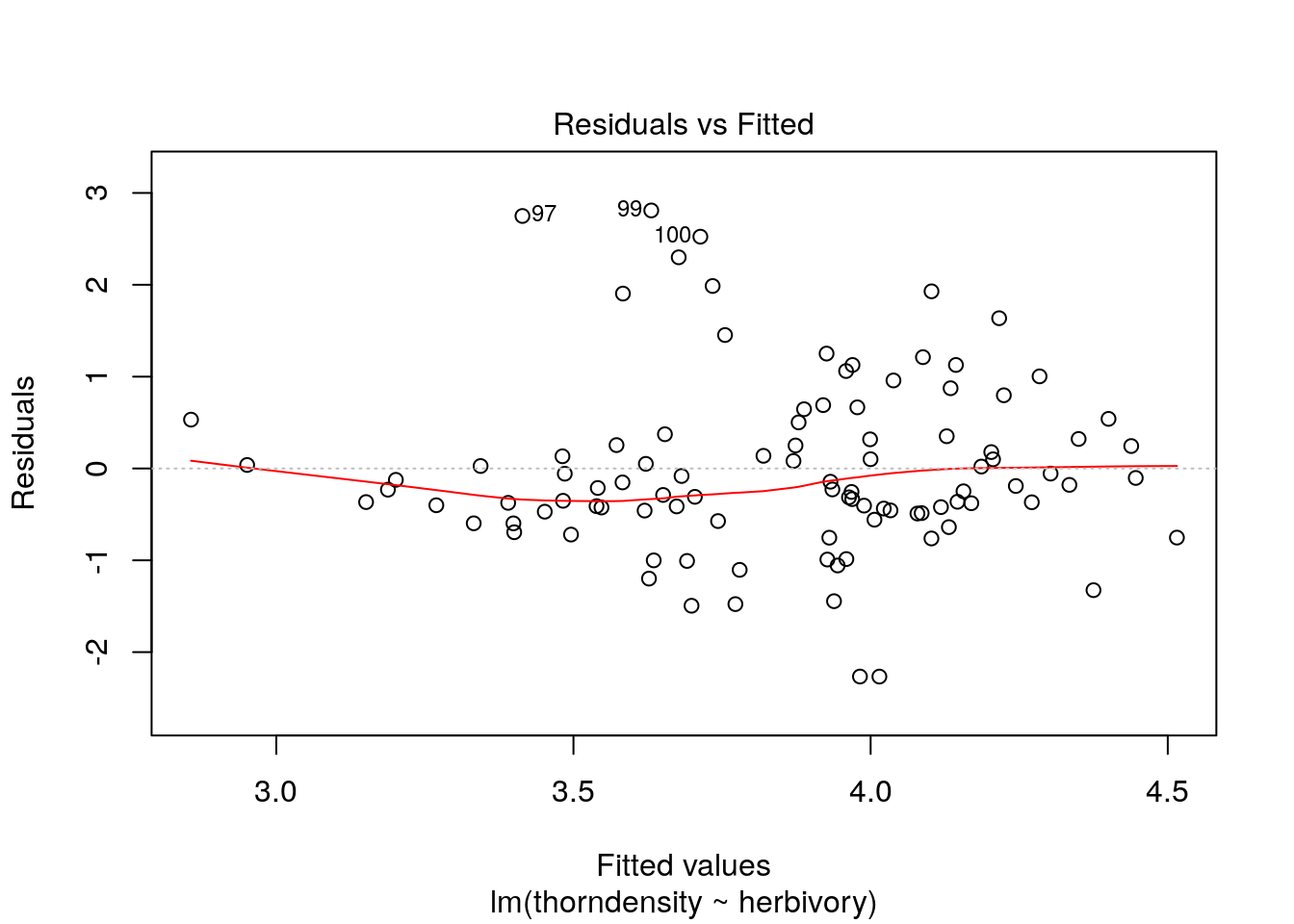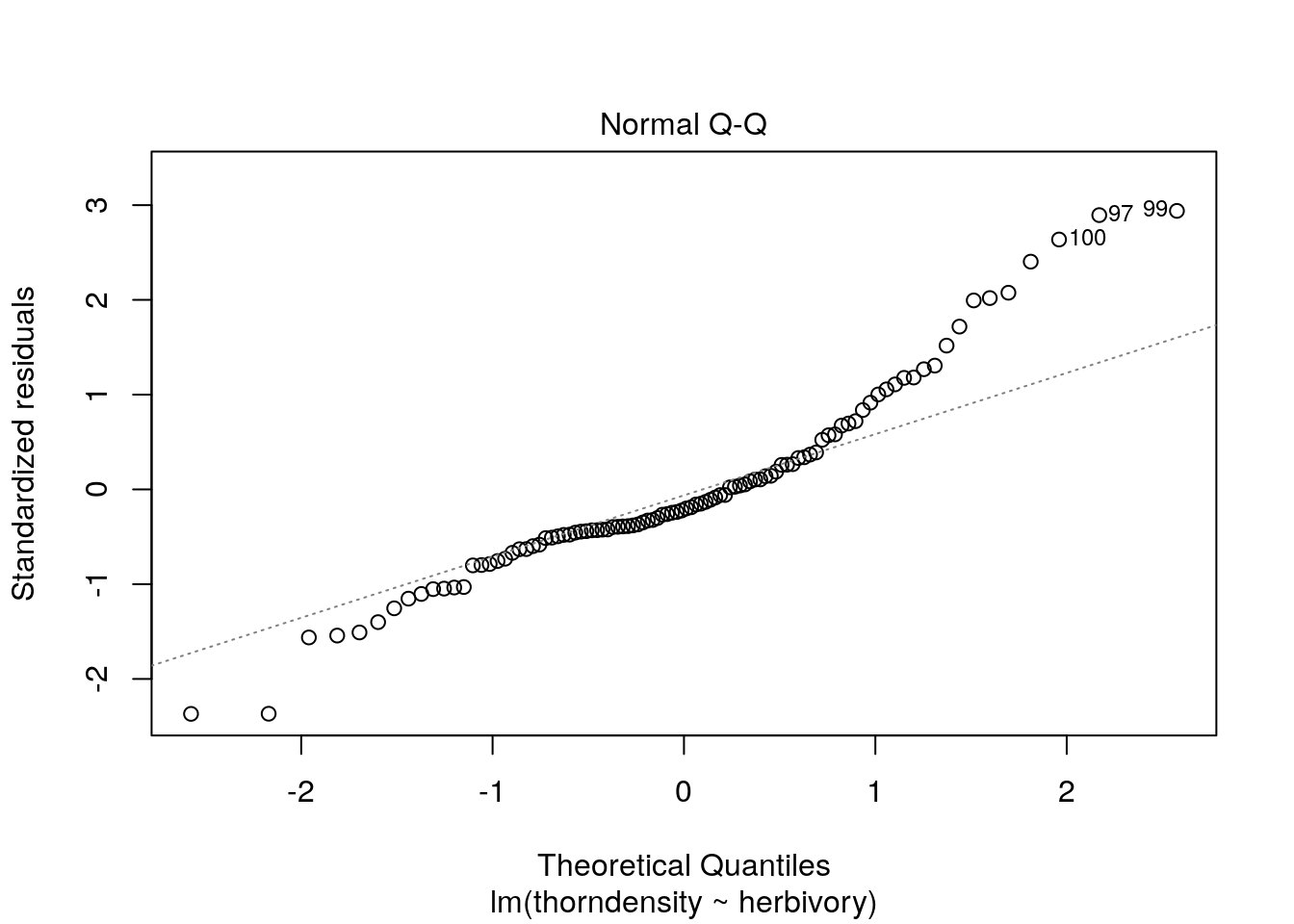``library(lmerTest)``
``## Loading required package: lme4``
``## Loading required package: Matrix``
``````##
## Attaching package: 'lmerTest'``````
``````## The following object is masked from 'package:lme4':
##
##     lmer``````
``````## The following object is masked from 'package:stats':
##
##     step``````
``library(ggplot2)``

Take-home:

• Not accounting for structure in the response variable can hide the true relationship between two variables
• Not accounting for structure in the response variable will make your models underestimate uncertainty.
• Use random effects to efficiently capture variation due to grouping factors and get better parameter estimates and more correct standard errors and p-values.

Imagine you have measured how much a plant species is attacked by big herbivores as a function of how many thorns the plant grows on stems. You have collected data in 5 locations and visualise the relationship between herbivory and quantity of thorns

``````thorndata <- read.csv("data/thorndata.csv")
str(thorndata)``````
``````## 'data.frame':    100 obs. of  3 variables:
##  \$ herbivory   : num  4.53 4.6 3.89 4.05 3.73 ...
##  \$ thorndensity: num  1.72 1.75 2.2 2.3 2.43 ...
##  \$ site        : Factor w/ 5 levels "a","b","c","d",..: 1 1 1 1 1 1 1 1 1 1 ...``````
``````ggplot(aes(x=thorndensity, y= herbivory), data = thorndata) +  geom_point()+
geom_smooth(method = "lm")``````
``## `geom_smooth()` using formula 'y ~ x'````````m0 <- lm(thorndensity ~ herbivory, data=thorndata)
summary(m0)``````
``````##
## Call:
## lm(formula = thorndensity ~ herbivory, data = thorndata)
##
## Residuals:
##     Min      1Q  Median      3Q     Max
## -2.2660 -0.4745 -0.2017  0.3563  2.8093
##
## Coefficients:
##             Estimate Std. Error t value Pr(>|t|)
## (Intercept)   1.9691     0.5428   3.628 0.000456 ***
## herbivory     0.4447     0.1264   3.520 0.000657 ***
## ---
## Signif. codes:  0 '***' 0.001 '**' 0.01 '*' 0.05 '.' 0.1 ' ' 1
##
## Residual standard error: 0.9627 on 98 degrees of freedom
## Multiple R-squared:  0.1122, Adjusted R-squared:  0.1032
## F-statistic: 12.39 on 1 and 98 DF,  p-value: 0.0006566``````
``plot(m0)``